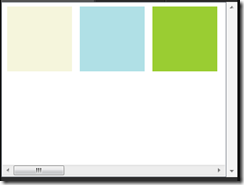I recently needed to put some items inside a `WrapPanel` in WPF, which I wanted to scroll vertically if there were too many items to fit into the available space. I was undecided on whether I wanted to use an `ItemsControl` or a `ListView` (which adds selected item capabilities), and discovered that when you switch between the two containers, the technique for getting the `WrapPanel` working is subtly different.

`ItemsControl` is the simplest. Just set the `ItemsPanelTemplate` to be a `WrapPanel`, and then put the whole thing inside a `ScrollViewer` (sadly you can’t put the `ScrollViewer` inside the `ItemsPanelTemplate`):

``````<ScrollViewer>
<ItemsControl>
<ItemsControl.ItemsPanel>
<ItemsPanelTemplate>
<WrapPanel />
</ItemsPanelTemplate>
</ItemsControl.ItemsPanel>
<Rectangle Margin="5" Width="100" Height="100" Fill="Beige" />
<Rectangle Margin="5" Width="100" Height="100" Fill="PowderBlue" />
<Rectangle Margin="5" Width="100" Height="100" Fill="#FF9ACD32" />
<Rectangle Margin="5" Width="100" Height="100" Fill="#FFFF6347" />
<Rectangle Margin="5" Width="100" Height="100" Fill="#FF6495ED" />
<Rectangle Margin="5" Width="100" Height="100" Fill="#FFFFA500" />
<Rectangle Margin="5" Width="100" Height="100" Fill="#FFFFD700" />
<Rectangle Margin="5" Width="100" Height="100" Fill="#FFFF4500" />
<Rectangle Margin="5" Width="100" Height="100" Fill="#FF316915" />
<Rectangle Margin="5" Width="100" Height="100" Fill="#FF8E32A7" />
<Rectangle Margin="5" Width="100" Height="100" Fill="#FFECBADC" />
<Rectangle Margin="5" Width="100" Height="100" Fill="#FFE6D84F" />
</ItemsControl>
</ScrollViewer>
``````

this produces the following results:But if you switch to an `ItemsView` instead, you’ll find that you get a single row of items with a horizontal scrollbar while the outer `ScrollViewer` has nothing to do.The solution is to disable the horizontal scrollbar of the `ListView` itself:

``````<ListView ScrollViewer.HorizontalScrollBarVisibility="Disabled">
``````

This allows our top-level `ScrollViewer` to work as with the `ItemsControl` and we have selection capabilities as well:The full XAML for the `ListView` with vertically scrolling WrapPanel is:

``````<ScrollViewer>
<ListView ScrollViewer.HorizontalScrollBarVisibility="Disabled">
<ListView.ItemsPanel>
<ItemsPanelTemplate>
<WrapPanel />
</ItemsPanelTemplate>
</ListView.ItemsPanel>
<Rectangle Margin="5" Width="100" Height="100" Fill="Beige" />
<Rectangle Margin="5" Width="100" Height="100" Fill="PowderBlue" />
<Rectangle Margin="5" Width="100" Height="100" Fill="#FF9ACD32" />
<Rectangle Margin="5" Width="100" Height="100" Fill="#FFFF6347" />
<Rectangle Margin="5" Width="100" Height="100" Fill="#FF6495ED" />
<Rectangle Margin="5" Width="100" Height="100" Fill="#FFFFA500" />
<Rectangle Margin="5" Width="100" Height="100" Fill="#FFFFD700" />
<Rectangle Margin="5" Width="100" Height="100" Fill="#FFFF4500" />
<Rectangle Margin="5" Width="100" Height="100" Fill="#FF316915" />
<Rectangle Margin="5" Width="100" Height="100" Fill="#FF8E32A7" />
<Rectangle Margin="5" Width="100" Height="100" Fill="#FFECBADC" />
<Rectangle Margin="5" Width="100" Height="100" Fill="#FFE6D84F" />
</ListView>
</ScrollViewer>
``````